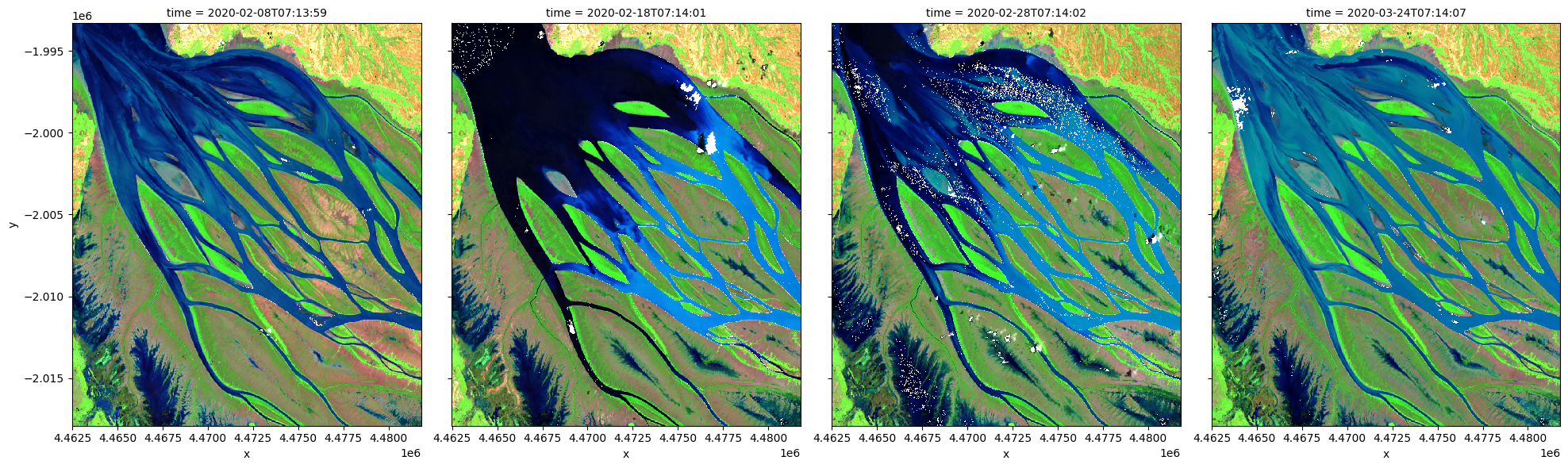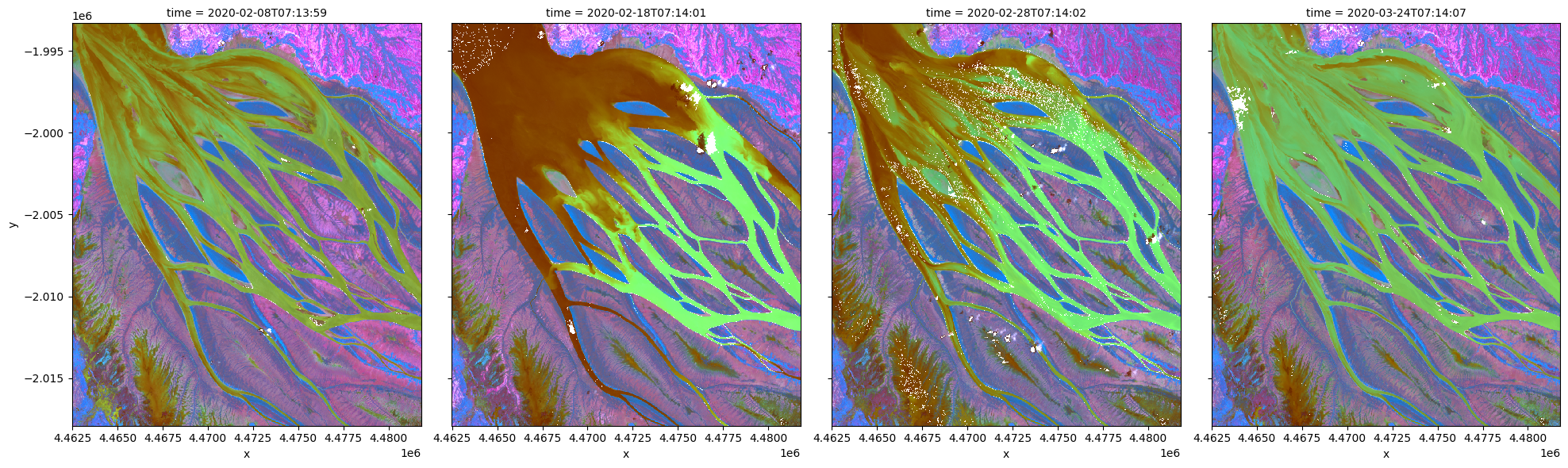# Principal component analysis for multi-spectral data¶

Keywords data used; sentinel-2, analysis; principal component analysis

## Background¶

Principal Component Analysis (PCA) is a popular technique for dimensionality reduction. It can be used to explore patterns in high-dimensional data and assist unsupervised learning.

Principal components are a series of linear combinations of the original variables, among which the first principal component accounts for the greatest variance within a dataset. Each subsequent principal component accounts for the next greatest possible variance and is uncorrelated with the previously defined components.

This technique is useful for understanding Sentinel-2 data as images are captured in 12 spectral bands but only 3 variables can be visualized in a RGB composite. PCA can also be applied to timeseries data to investigate temporal evolution patterns for different land cover types.

## Description¶

This notebook demonstrates a principal component analysis for Sentinel-2 multi-spectal data. Following steps are covered:

2. Applying PCA to transform and visualize data. ***

## Getting started¶

To run this analysis, run all the cells in the notebook, starting with the “Load packages” cell.

Import Python packages that are used for the analysis.

:

%matplotlib inline

from sklearn.decomposition import PCA
import datacube

from deafrica_tools.plotting import rgb
from deafrica_tools.classification import sklearn_flatten, sklearn_unflatten

/env/lib/python3.8/site-packages/datacube/storage/masking.py:7: DeprecationWarning: datacube.storage.masking has moved to datacube.utils.masking
/env/lib/python3.8/site-packages/geopandas/_compat.py:106: UserWarning: The Shapely GEOS version (3.8.0-CAPI-1.13.1 ) is incompatible with the GEOS version PyGEOS was compiled with (3.9.1-CAPI-1.14.2). Conversions between both will be slow.
warnings.warn(


### Connect to the datacube¶

Connect to the datacube so we can access DEAfrica data.

:

dc = datacube.Datacube(app='pca')


### Analysis parameters¶

This section defines the analysis parameters, including

• center lat/lon and analysis window size for the area of interest

• time period to be investigated

• acceptable range of cloud cover percentage for input Sentinel-2 granule (min_gooddata)

• spectral bands to be explored

The default location is Betsiboka Estuary, Madagascar.

To limit overall memory usage, if a larger analysis window or higher resolution is desired, the time period should be reduced accordingly.

:

# Create a query object
lat, lon = -15.92, 46.35
buffer = 0.1

query = {
'time': ('2020-01', '2020-03'),
'x': (lon - buffer, lon + buffer),
'y': (lat + buffer, lat - buffer),
'output_crs': 'epsg:6933',
'resolution':(-20,20),
}

# use all non-overlapping 10m and 20m bands
bands = ['blue', 'green', 'red', 'red_edge_1', 'red_edge_2',
'red_edge_3', 'nir_narrow', 'swir_1', 'swir_2']


Only high probablity cloud is excluded in this example, but this can be modified for a different area.

:

ds = load_ard(dc=dc,
products=['s2_l2a'],
measurements=bands,
min_gooddata=0.98,
group_by='solar_day',
**query)

Using pixel quality parameters for Sentinel 2
Finding datasets
s2_l2a
Counting good quality pixels for each time step
Filtering to 4 out of 18 time steps with at least 98.0% good quality pixels

:

# visualize data using selected input spectral bands
rgb(ds, bands=['swir_1','nir_narrow','red_edge_1'], index=list(range(len(ds.time))), col_wrap=4)## Applying PCA to transform and visualize data¶

To perform a PCA, data is first transformed into a numpy array that can be used by sklearn.

:

X = sklearn_flatten(ds)


A PCA model is generated with 3 principal components and fitted on the data.

:

pca = PCA(n_components=3)
pca.fit(X)

:

PCA(n_components=3)


We can investigate how much variance is accounted for in each principal component. In the default example, the first principal component accounts for a much high variance than the next two.

This step can help determine whether more principal components are needed.

:

print("Relative variance in principal components:", pca.explained_variance_ratio_)

Relative variance in principal components: [0.63061713 0.21630557 0.14659838]


The input data can now be transformed into this new reference space and rearranged into a xarray dataset compatible with input data.

:

predict = pca.transform(X)

:

out = sklearn_unflatten(predict, ds)
out = out.to_dataset(dim=out.dims).transpose('time','y','x')


### Visualise PCA results¶

:

rgb(out, bands=[2,1,0], index=list(range(len(out.time))), col_wrap=4)Contact: If you need assistance, please post a question on the Open Data Cube Slack channel or on the GIS Stack Exchange using the open-data-cube tag (you can view previously asked questions here). If you would like to report an issue with this notebook, you can file one on Github.

Compatible datacube version:

:

print(datacube.__version__)

1.8.5


Last Tested:

:

from datetime import datetime
datetime.today().strftime('%Y-%m-%d')

:

'2021-09-13'